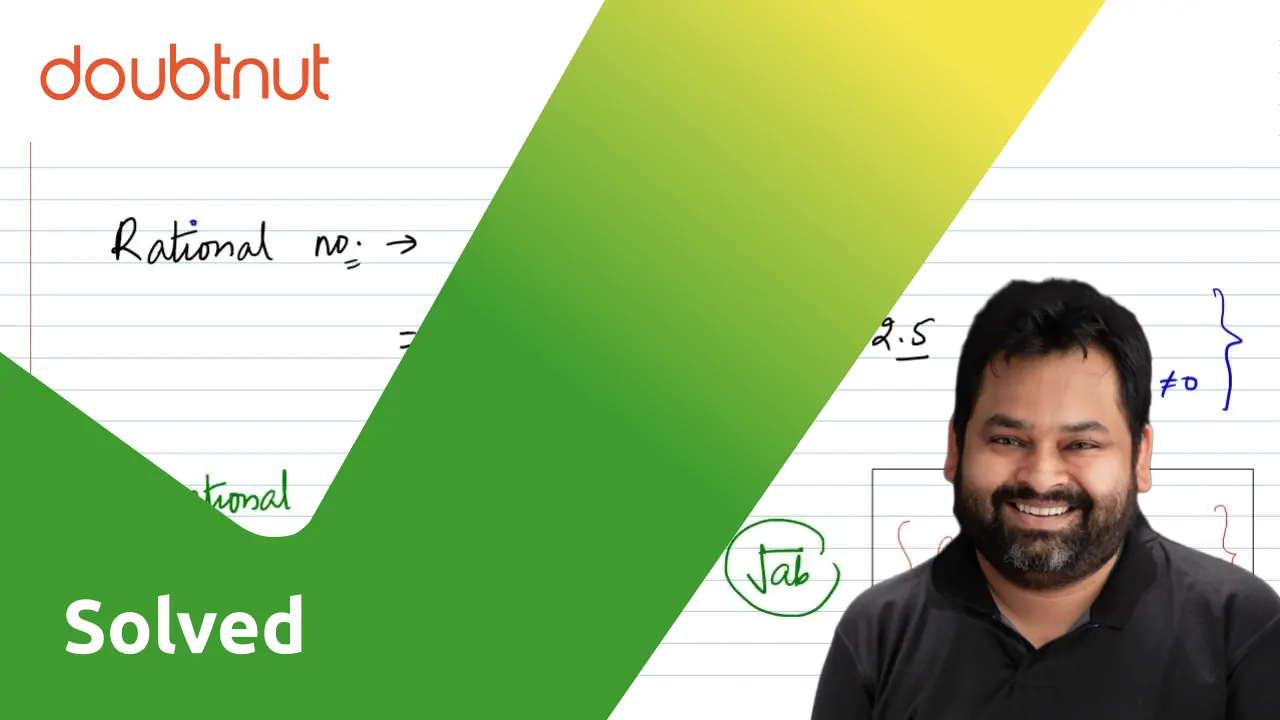Doubtnut App - Get 50 Lakh+ video solutions better than GoogleInstall Now

# Insert a rational and irrational number between 2 and 3

Text Solution
Verified by Experts

## There are infinite rational numbers between two rational numbers. if a and b are the two rational numbers then rational number between them can be a+b2 and irrational number is √ab provided ab is not perfect square here a=2andb=3 the 1 rational number is - 52 Now irrational number=√6